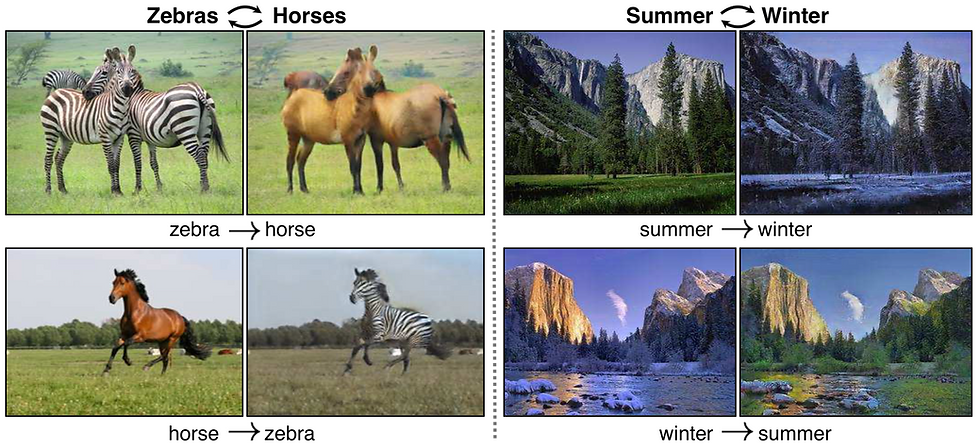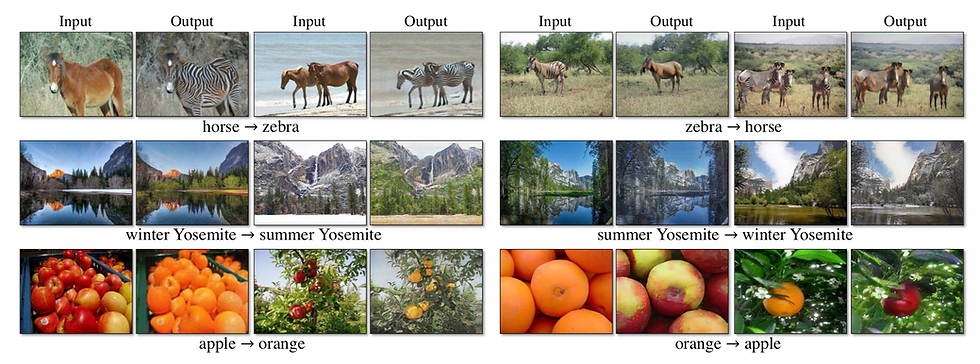top of page
Search
• Xuehao Liu

# cycleGAN image translate

Updated: Mar 14, 2019In many image translation problem, the training dataset is paired. For example, if you want to translate a satellite map to a normal map, or you want to translate edge doodles to photos, you need exactly the same pair of two maps to train.This image pair is hand-picked and it is expensive.

cycleGAN is designed to use unpaired datasets to train the network that can do the same translate work. As the example shown above, we just have the dataset which only has the labels of two classes. We know this image is a photo, and the other one is a painting. That is all we know. We do not have the correspondence of each instance. And we do not need to know.

The concept of mapping

The main target is to find the mapping between X to Y. For example, we want to change a horse to a zebra. There must be a mapping relationship between X and Y(horse and zebra). If there is a horse from X, there must be a zebra version of that horse in Y, and vise versa. If we use these two categories to train cycleGAN, we assume this mapping exists.

StructureThis is the structure of cycleGAN. It has many similar aspects with the pix2pix GAN structure. But cycleGAN is a bit more complex. The idea of the GAN is to use a generative network(G) to generate results and at the same time use another discriminator(D) to supervise the results from G. Both G and D are trained together. cycleGAN takes one step further. It has two generative networks(G and F). G is responding to generate fake Y(Y'). And F is responding to generate fake X(X'). There are also two discriminators(Dx and Dy). Dx will test whether the input is a real X, and Dy will do the same test on Y. With Dx, Dy, G and F, we can build cycles.

The first cycle is x -> y' -> x'. We can use G with any x in X to generate a fake Y, y'. And use F and this y' to generate another fake X, x".

The second cycle is y -> x' -> y'. We can use F with any y in Y to generate a fake X, x'. And use G and this x' to generate another fake Y, y".

You see these two cycles are basically the same thing.

Loss function

If we need to train these four networks we need a loss function. The first question is: What we want?

We need everything perfect.

If G and F are the perfect generative networks. The fake X(x') and fake Y(y') are no longer fake. They are real X and Y. And when we translate them back, x" and y" should be the original x and y, because G and F are perfect! So we can calculate the distance between x and x", y and y".

If Dx and Dy are perfect discriminators. They will be able to say: only x and y are real. x', x", y', and y" are all fake. So we can give these six outputs labels and calculate the classification loss.

Train strategy

One thing needs to be mentioned of training cycleGAN is that these four networks are trained at the same time in every iteration.

Structure of G and F

G and F are the same generators in pix2pix. It is a three-layer encoder + nine residual blocks + three-layer decoder.

Structure of Dx and Dy

Dx and Dy are Markovian discriminators. The normal discriminators are the normal CNNs, which have five or six convolutional layers. The output of the normal discriminator has one dimension. It decides whether the input is real.

The Markovian discriminators are just doing the convolution on a small window on the image. This small window is randomly selected. Normally it is 70*70. The rest layers are the same.

Obviously the Markovian discriminators have less computing burden. And in the actual experiments, the Markovian discriminators did not make the results worse.

Fake images pool

The paper also introduced an additional way to make the discriminator more generalize. It will maintain two fake pools for two kinds of fake images. At the beginning of the training, the pool is empty. As the training process goes on, the pool will be filled by generated images. After the first iteration, the discriminator will randomly pick one instance from the fake pool and add it to the loss function. This makes the discriminator can see the instances from the past.

More result examples of cycleGAN:The results in the paper also shows that the results of the network can deceive human testers in some level. It is better than many other old GAN networks.

So you may say the results looks not bad. Does it always work?This is a failure example from the paper. The man on the horse is changed to zebra as well. The reason from the paper is that the network has never seen an example that a man is riding a horse in the training process. This is interesting. It makes people wondering: what is the boundary of using cycleGAN to translate images? What is the actual limitation for this method? And moreover, if we have the dataset which has the instances of a man riding a horse or zebra, can we train a network to do the translation which can just change the horse to zebra and keep the man intact?

Anyway, this is a powerful network and it has proved that it is a valid method to the image translation.